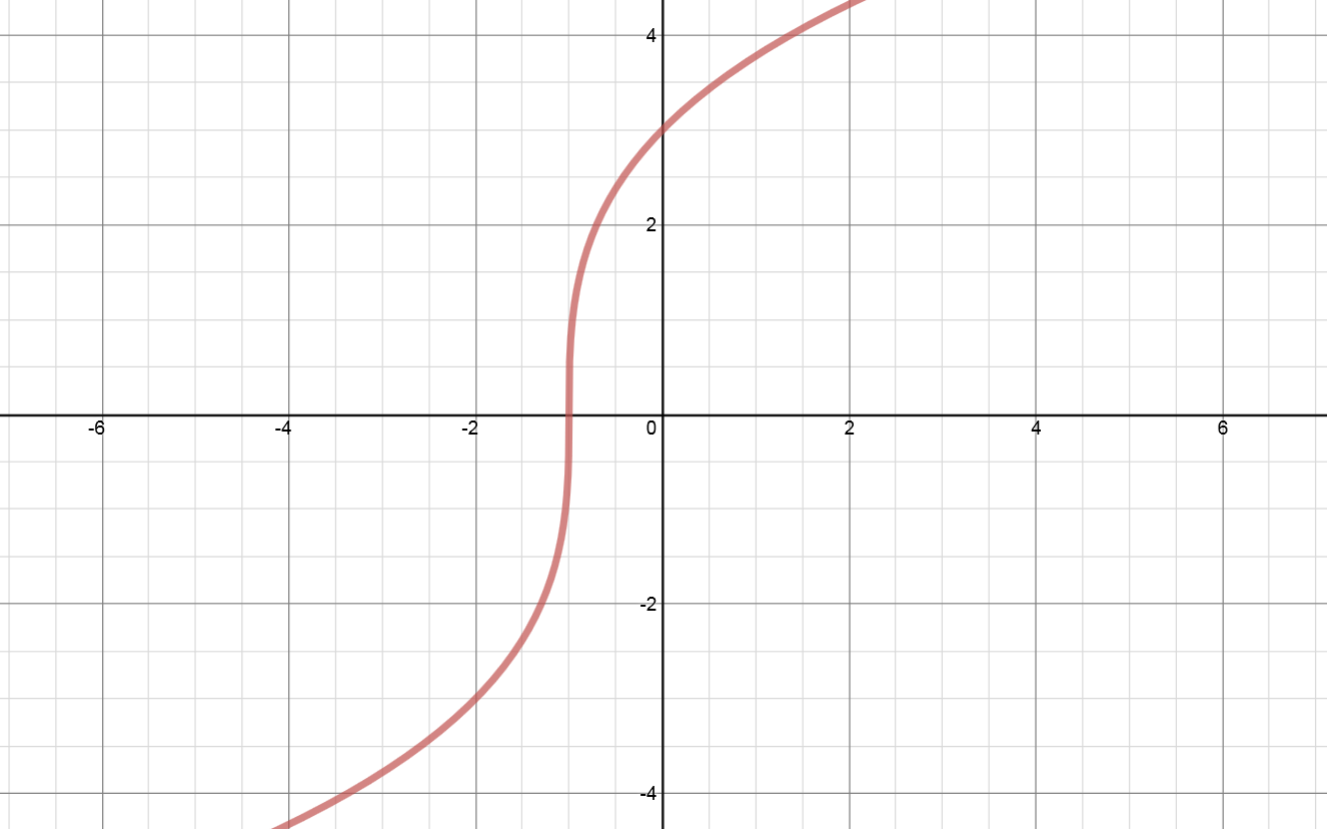# Transformations of functions: Horizontal stretches - Relations and Functions

### Transformations of functions: Horizontal stretches

#### Lessons

##### In conclusion: • $\left( x \right) \to \left( {2x} \right)$: horizontal stretch by a factor of ________ ⇒ all $x$ coordinates ______________________. • $\left( x \right) \to \left( {\frac{1}{3}x} \right)$: horizontal stretch by a factor of ________ ⇒ all $x$ coordinates ______________________.